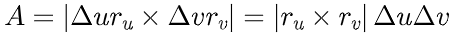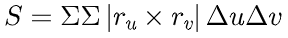# Estimating the area of a surface

Author:
Art Busch
Topic:
Area, Surface
• Fixed values of the parameters u and v determine a point Q on the surface.
• Fixing u but not v creates a parametric curve through Q that lies on the surface, and fixing v but not u creates another curve on the surface through Q.
• Choosing two small step sizes defines two additional curves that enclose a small region on the surface.
• By using the step sizes to scale the tangent vectors, we can define a parallelogram in the plane tangent to S at Q.
• The area of that parallelogram closely approximates the area of the enclosed region on the surface.
• We can calculate the area of this parallelogram using cross products:

﻿﻿• Summing over all the "cells" of the surface gives an estimate of the total surface ares:﻿• Taking the limit as Δu and Δv go to zero gives: